ORDERS OF REACTION AND RATE EQUATIONS

```
```

Changing the concentration of substances taking part in a reaction usually changes the rate of the reaction. A rate equation shows this effect mathematically. Orders of reaction are a part of the rate equation. This page introduces and explains the various terms you will need to know about.

Note:  If you aren't sure about why changing concentration affects rates of reaction you might like to follow this link and come back to this page afterwards - either via the rates of reaction menu or by using the BACK button on your browser.

Rate equations

Measuring a rate of reaction

There are several simple ways of measuring a reaction rate. For example, if a gas was being given off during a reaction, you could take some measurements and work out the volume being given off per second at any particular time during the reaction.

A rate of 2 cm3 s-1 is obviously twice as fast as one of 1 cm3 s-1.

Note:  Read cm3 s-1 as "cubic centimetres per second".

However, for this more formal and mathematical look at rates of reaction, the rate is usually measured by looking at how fast the concentration of one of the reactants is falling at any one time.

For example, suppose you had a reaction between two substances A and B. Assume that at least one of them is in a form where it is sensible to measure its concentration - for example, in solution or as a gas.For this reaction you could measure the rate of the reaction by finding out how fast the concentration of, say, A was falling per second.

You might, for example, find that at the beginning of the reaction, its concentration was falling at a rate of 0.0040 mol dm-3 s-1.

Note:  Read mol dm-3 s-1 as "moles per cubic decimetre (or litre) per second".

This means that every second the concentration of A was falling by 0.0040 moles per cubic decimetre. This rate will decrease during the reaction as A gets used up.

Summary

For the purposes of rate equations and orders of reaction, the rate of a reaction is measured in terms of how fast the concentration of one of the reactants is falling. Its units are mol dm-3 s-1.

```
```

Orders of reaction

I'm not going to define what order of reaction means straight away - I'm going to sneak up on it!

Orders of reaction are always found by doing experiments. You can't deduce anything about the order of a reaction just by looking at the equation for the reaction.

So let's suppose that you have done some experiments to find out what happens to the rate of a reaction as the concentration of one of the reactants, A, changes. Some of the simple things that you might find are:

One possibility: The rate of reaction is proportional to the concentration of A

That means that if you double the concentration of A, the rate doubles as well. If you increase the concentration of A by a factor of 4, the rate goes up 4 times as well.

You can express this using symbols as:Writing a formula in square brackets is a standard way of showing a concentration measured in moles per cubic decimetre (litre).

You can also write this by getting rid of the proportionality sign and introducing a constant, k.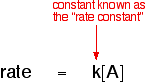```
```

Another possibility: The rate of reaction is proportional to the square of the concentration of A

This means that if you doubled the concentration of A, the rate would go up 4 times (22). If you tripled the concentration of A, the rate would increase 9 times (32). In symbol terms: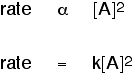```
```

Generalising this

By doing experiments involving a reaction between A and B, you would find that the rate of the reaction was related to the concentrations of A and B in this way: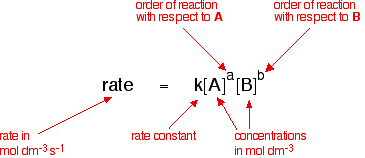This is called the rate equation for the reaction.

The concentrations of A and B have to be raised to some power to show how they affect the rate of the reaction. These powers are called the orders of reaction with respect to A and B.

For UK A' level purposes, the orders of reaction you are likely to meet will be 0, 1 or 2. But other values are possible including fractional ones like 1.53, for example.

If the order of reaction with respect to A is 0 (zero), this means that the concentration of A doesn't affect the rate of reaction. Mathematically, any number raised to the power of zero (x0) is equal to 1. That means that that particular term disappears from the rate equation.

The overall order of the reaction is found by adding up the individual orders. For example, if the reaction is first order with respect to both A and B (a = 1 and b = 1), the overall order is 2. We call this an overall second order reaction.

Some examples

Each of these examples involves a reaction between A and B, and each rate equation comes from doing some experiments to find out how the concentrations of A and B affect the rate of reaction.

Example 1: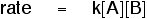In this case, the order of reaction with respect to both A and B is 1. The overall order of reaction is 2 - found by adding up the individual orders.

Note:  Where the order is 1 with respect to one of the reactants, the "1" isn't written into the equation. [A] means [A]1.

Example 2: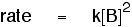This reaction is zero order with respect to A because the concentration of A doesn't affect the rate of the reaction. The order with respect to B is 2 - it's a second order reaction with respect to B. The reaction is also second order overall (because 0 + 2 = 2).

Example 3: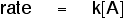This reaction is first order with respect to A and zero order with respect to B, because the concentration of B doesn't affect the rate of the reaction. The reaction is first order overall (because 1 + 0 = 1).

What if you have some other number of reactants?

It doesn't matter how many reactants there are. The concentration of each reactant will occur in the rate equation, raised to some power. Those powers are the individual orders of reaction. The overall order of the reaction is found by adding them all up.

```
```

The rate constant

Surprisingly, the rate constant isn't actually a true constant! It varies, for example, if you change the temperature of the reaction, add a catalyst, or change the catalyst.

The rate constant is constant for a given reaction only if all you are changing is the concentration of the reactants. You will find more about the effect of temperature and catalysts on the rate constant on another page.

Note:  If you want to follow up this further look at rate constants you might like to follow this link. Alternatively, you could visit it later via the rates of reaction menu.

Calculations involving orders of reaction

You will almost certainly have to be able to calculate orders of reaction and rate constants from given data or from your own experiments.

There are all sorts of ways of doing these sums, and it is important that you practice the methods that your syllabus wants. Check your syllabus and past exam papers to see what sort of examples you need to be able to work out.

Note:  For UK A'level students, if you haven't got copies of your syllabus and past papers follow this link to find out how to get hold of them.

Many text books make these sums look really difficult. In fact for A' level purposes, the calculations are usually fairly trivial. You will find them explained in detail in my chemistry calculations book.

Note:  There are several reasons why there are very few calculations on this site. It is much easier to learn to do sums from a carefully organised book than from a website; I would be in breach of my contract with my publishers if I included material similar to what is in the book; and I need to sell a few books to generate some income!

If you are interested in my chemistry calculations book you might like to follow this link.

```
```
 Questions to test your understanding If this is the first set of questions you have done, please read the introductory page before you start. You will need to use the BACK BUTTON on your browser to come back here afterwards. questions on orders or reaction answers
```
```

Where would you like to go now?

To a simple look at how orders of reaction are related to reaction mechanisms . .

To the rates of reaction menu . . .

To the Physical Chemistry menu . . .

To Main Menu . . .

```
```

© Jim Clark 2002 (modified October 2013)